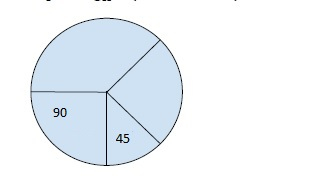# Program to find smallest difference of angles of two parts of a given circle in C++

In this problem, we are given an array that denotes the piece of a circle based on the angles of a circle. Our task is to create a program to find smallest difference of angles of two parts of a given circle in C++.

Problem Description − We are given the angles of all pieces of the circle in the array. We need to join the piece in such a way that the two pieces made have the smallest difference of angle.

Let’s take an example to understand the problem

## Input

ang[] = {90, 45, 90, 135}## Output

90

## Explanation

Taking 1st and 2nd together i.e. 90 + 45 = 135.

Taking 3rd and 4th together i.e. 90 + 135 = 225

Difference = 225 - 135 = 90

## Solution Approach

Here, we have to merge all parts to make two parts. And for that, we need to take continuous parts (in the example we can’t take ang1 and ang3 together).

Let’s take the angle of part1 be A.

Then the angle of part2 will be 360 - A.

The difference will be |A - (360 - A)|. We have taken absolute value as there can only be positive angles.

Solving the difference equation,

2 * |A - 180|, this needs to be the minimum value. And for that, we will try to merge all possible parts of the circle and find the minimum value for (2* |A - 180|).

Program to illustrate the working of our solution

## Example

Live Demo

#include <iostream>
#include <math.h>
using namespace std;
int CalcSmallDiffAng(int ang[], int n) {
int Left = 0, A = 0, minDiff = 360;
for (int i = 0; i < n; i++) {
A += ang[i];
while (A >= 180) {
minDiff = min(minDiff, 2 * abs(180 - A));
A -= ang[Left];
Left++;
}
minDiff = min(minDiff, 2 * abs(180 - A));
}
return minDiff;
}
int main() {
int ang[] = { 90, 45, 90, 135 };
int n = sizeof(ang) / sizeof(ang);
cout<<"The smallest difference of angles of two parts of a given
circle is "<<CalcSmallDiffAng(ang, n);
return 0;
}

## Output

The smallest difference of angles of two parts of a given circle is 90